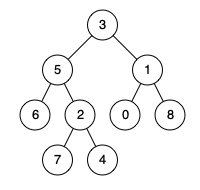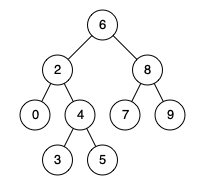## 236. Lowest Common Ancestor of a Binary Tree

### Description

Given a binary tree, find the lowest common ancestor (LCA) of two given nodes in the tree.

According to the definition of LCA on Wikipedia: “The lowest common ancestor is defined between two nodes p and q as the lowest node in T that has both p and q as descendants (where we allow a node to be a descendant of itself).”

Given the following binary tree: root = [3,5,1,6,2,0,8,null,null,7,4]Example 1:

Example 2:

Note:

• All of the nodes’ values will be unique.
• p and q are different and both values will exist in the binary tree.

### Some test cases

• neither p nor q is the LCA
• either p or q is the LCA
• single node tree
• null node
• p and q are the same node (eliminated by problem statement, follow up 3)
• either p or q are null (eliminated by problem statement, follow up 3)
• either p or q not exists in tree (eliminated by problem statement, follow up 3)

### DFS recursive

The recursive dfs approach is straight forward. The time complexity is $O(N)$ since we are traversing each node of the entire tree to find match with p and q. The space complexity is the height of the recursive tree which is $O(logN)$.

#### DFS recursive Algorithm

There are two cases. One case where neither p or q is the LCA. The other case is when either p or q is the LCA. Since the default return type is TreeNode. Lets

• return root if root == null
• return root if p == root or q == root
• return root if left != null && right != null.
• return left if left != null.
• Other wise, return right.

Bottom-up approach also work, but might be less optimzied than version above.

A slight micro-optization for recursive dfs version with 2 if statement checks (intead of 3 checks) in best case.

### DFS iterative

Iterative DFS with a stack allow tree traversal, however, we still need a way to know node’s parent to find the shared LCA. Hence, as we traversing the tree to find p and q, we also need to record [child -> parent] relation along the way. Time complexity of this algorithm is $O(N)$ in worst case and space complexity is $O(N)$ for iterative stack and the hashmap for storing <child, parent> relation.

#### DFS iterative Algorithm

• traverse tree itertively with stack to look for p and q
• use HashMap<TreeNode, TreeNode> parent to record <child, parent> relation.
• once both p and q found (child, parent relation for both p and q found)
• add p‘s all ancester to a Set
• traverse q‘s ancesters in order, and first shared ancester is the shared LCA

## [Followup 1] 235. Lowest Common Ancestor of a Binary Search Tree

### Description

Given a binary search tree (BST), find the lowest common ancestor (LCA) of two given nodes in the BST.

According to the definition of LCA on Wikipedia: “The lowest common ancestor is defined between two nodes p and q as the lowest node in T that has both p and q as descendants (where we allow a node to be a descendant of itself).”

Given binary search tree: root = [6,2,8,0,4,7,9,null,null,3,5]> Solve problem here

Example 1:

Example 2:

Note:

• All of the nodes’ values will be unique.
• p and q are different and both values will exist in the BST.

### Thought

Since it is a BST, we start from root of the tree, if p.val & q.val are both smaller than root.val, p and q are in the left subtree. If p.val & q.val are both larger than root.val, both node are at right subtree. Otherwise, root is the lowest ancester. (last ancester).

Beacuse the special structure of BST, we only need to visit at most $log(N)$ node along the way. Hence, the time complexity is $O(log N)$. The space complexity is $O(log N)$ for height of the recursive stack.

### Iterative w/ queue

Time: $O(logN)$ and Space: $O(logN)$

### Iterative wo/ queue [preferred]

Time: $O(logN)$ and Space: $O(1)$

## [Followup 2] 474. Lowest Common Ancestor II

### Description

Given the root and two nodes in a Binary Tree. Find the lowest common ancestor (LCA) of the two nodes. The lowest common ancestor is the node with largest depth which is the ancestor of both nodes. The node has an extra attribute parent which point to the father of itself. The root’s parent is null.

> Solve problem here

Example

Example 1:

Example 2:

### Thought

The follow up problem provides extra parent pointer for each TreeNode. With this, we can eliminate the time requires to traverse the entire tree to locate p and q node. Instead, we can trace directly from p node to root and add all its ancester to a Set in ordder. Then trace from q to root and return the first shared node as LCA

## [Followup 3] 578. Lowest Common Ancestor III

Description

Given the root and two nodes in a Binary Tree. Find the lowest common ancestor(LCA) of the two nodes.
The lowest common ancestor is the node with largest depth which is the ancestor of both nodes.
Return null if LCA does not exist.

Note: node A or node B may not exist in tree.

Example

For the following binary tree:

In this followup question

• node A or node B may not exist in tree
• node A and node B might also be the same node

It is very similiar to the original problem by adding only two line of code with two extra boolean varaibles foundA and foundB and some logic to record if both nodes were found. Time complxity $O(N)$ and space complexity is the recursive stack $O(logN)$

## Summary

• recursive dfs
• iterative dfs
• idea of top-down and bottom-up
• generate own parent pointer / given parent’s pointer
• what to change if p, and q not guarntee exists in tree